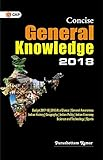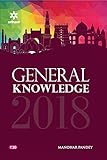# Principles of voltage, current & resistance measurement – Notes – 1 – 1

1. Analog meters use a graduated scale with a pointer.

2. Digital meters provide a direct readout.

3. On both analog and digital meters, the red terminal is positive and the black terminal is negative.

4. Before using an analog meter, check the mechanical zero adjustment.

5. A multimeter combines a voltmeter, ammeter, and ohmmeter into one package.

6. A VOM is an analog multimeter that measures volts, ohms, and milliamperes.

7. A DMM is a digital multimeter.

8. On a multimeter, the range selector switch selects the function to be used.

9. An ammeter must be connected in series with a circuit.

10. A voltmeter is connected in parallel with a circuit.

11.  An ohmmeter measures resistance by the amount of current flowing through the resistor being measured.

12. The maximum value of a meter scale is called the full scale value.

13. The number of divisions on the meter scale depends on the range the meter is designed to measure.

14. Ammeters and voltmeters are read from left to right and have a linear scale.

15. Ohmmeters are read from right to left and have nonlinear scales.

16. An analog ohmmeter must be calibrated before use to compensate for battery deterioration.

 Principles of voltage, current & resistance measurement – Mcq Principles of voltage, current & resistance measurement – Notes Principles of voltage, current & resistance measurement – IQ

General Knowledge Books##General Knowledge 2019 Price: INR 30.00-17% General Knowledge Price: INR 179.00 Was: INR 216.00-43% Objective General Knowledge Chapterwise Collection of 6250+ Questions Price: INR 169.00 Was: INR 295.00Concise General Knowledge 2018 Price: INR 30.00General Knowledge 2018 (Old Edition) Price: Check on Amazon-16% Objective General Knowledge Price: INR 302.00 Was: INR 360.00 ‹›

Be a Part of the New & Next

Share the Knowledge

Copy Protected by Chetan's WP-Copyprotect.